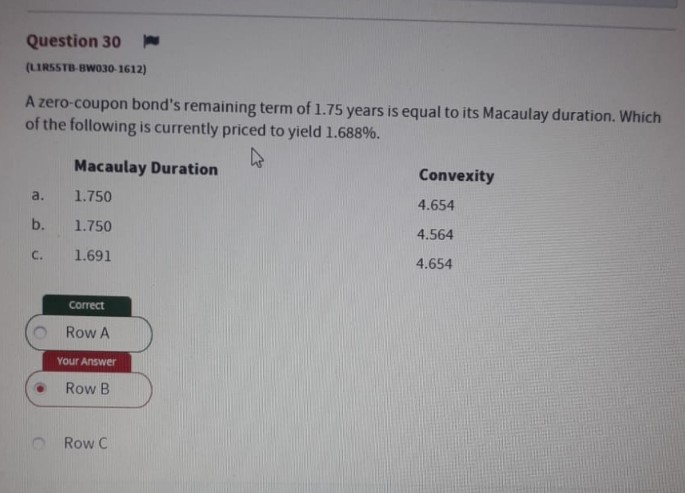# Can anyone explain the reasoning behind this fixed income question?key point is macaylay duration equals to its maturity when it’s a zero coupon because there is no pv for each payment during the time period except itself.
Then convexity formula makes you to find out PV+(interstate rate increase) and PV-(interest rate decrease), but you don’t have PVnull so need to calculate.
which calculate from the zero-coupon bond(N=1.75(given), PMT=0(cuz zero-coupon), FV=100(cuz par value), I/Y=1.688(given), PV=CPT),
then assume whatever the change rate you like to use, I like to use 10 bps, then you have (N=1.75, PMT=0, FV=0, I/Y for 1.588 and 1.688, PV= CPT for each PV+ and PV-)
then use the convexity formula you would have approximately 6.50